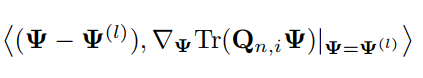# The problem about the DC programming

In the problem, rate is as follows

where psi is the variable.It’s a matrix.
In the (l+1)-th (l = 0, 1, . . . ) iteration of the DC programming, the second concave item is

I wonder how this term can be described in code.My idea is that this should not be a matrix, but < > should be matrix multiplied by matrix, but it’s still a matrix. How should I write it in code?

It’s your model (meaning, you extracted it from a book or paper), not ours. So it is your responsibility to understand what the notation means. That has nothing to do with CVX. And even though DC Programming might be used in the solution of this model, your question doesn’t have anything to do with DC Programming.

Nevertheless, in this context, `<>` likely means trace inner product. Specifically,
`<A.B> = trace(A'*B)`. If `A` is symmetric (hermitian), this is `<A.B> = trace(A*B)`.